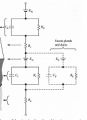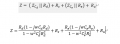# how can i calculate impedance

#### kareno

Joined Apr 14, 2020
4I want to find the impedance in this circuit.
Is it possible to calculate the capacitor as 1 / jwc after neglecting the voltage from this circuit?so I calculated it my way, it came out like a picture.(without dotted part)
If I use w instead of 2*pi*f, I am wondering this is correct.

Thank you for letting me know how to do the calculations with and without the dashed part of the circuit

#### WBahn

Joined Mar 31, 2012
25,776
Impedance as seen between what two terminals?

Your imagine is too low resolution to make out details.

#### WBahn

Joined Mar 31, 2012
25,776
It's correct depending one why you are ignoring the voltage source. Are you looking for large signal or small signal impedance?

#### kareno

Joined Apr 14, 2020
4
It's correct depending one why you are ignoring the voltage source. Are you looking for large signal or small signal impedance?
I want large signal impedance
that calculation is right for large signal?
if that isnt, how can i calculate impedance?

#### WBahn

Joined Mar 31, 2012
25,776
I want large signal impedance
that calculation is right for large signal?
if that isnt, how can i calculate impedance?
Large signal impedance is the relationship

Z(jω) = V(jω)/I(jω)

Ask yourself, if you apply some voltage V(jω) across that circuit, can you calculate I(jω) by taking that V(jω) and dividing it by I(jω)?

To answer that question, consider a very simple circuit.

I take a black box and inside it between the two terminals I put a 10 V battery in series with a 1 kΩ resistor.

If you say that the large signal impedance is 1 kΩ, then you are claiming that if someone takes that black box and connects a 12 V battery across it that 12 mA of current will flow? Is that the case? In fact, the amount of current that flows will depend on whether which way you connect the 12 V battery? But impedance isn't directional, so does talking about a large signal impedance for that black box make any sense at all?

#### kareno

Joined Apr 14, 2020
4
Large signal impedance is the relationship

Z(jω) = V(jω)/I(jω)

Ask yourself, if you apply some voltage V(jω) across that circuit, can you calculate I(jω) by taking that V(jω) and dividing it by I(jω)?

To answer that question, consider a very simple circuit.

I take a black box and inside it between the two terminals I put a 10 V battery in series with a 1 kΩ resistor.

If you say that the large signal impedance is 1 kΩ, then you are claiming that if someone takes that black box and connects a 12 V battery across it that 12 mA of current will flow? Is that the case? In fact, the amount of current that flows will depend on whether which way you connect the 12 V battery? But impedance isn't directional, so does talking about a large signal impedance for that black box make any sense at all?
Then, since there is a dc power supply in that circuit, can i calculate the power supply as a short circuit without having to worry about w?

#### WBahn

Joined Mar 31, 2012
25,776
Then, since there is a dc power supply in that circuit, can i calculate the power supply as a short circuit without having to worry about w?
That would be claiming that changing the frequency of the signal has no effect on the current for the same voltage applied. Do you believe that's true?